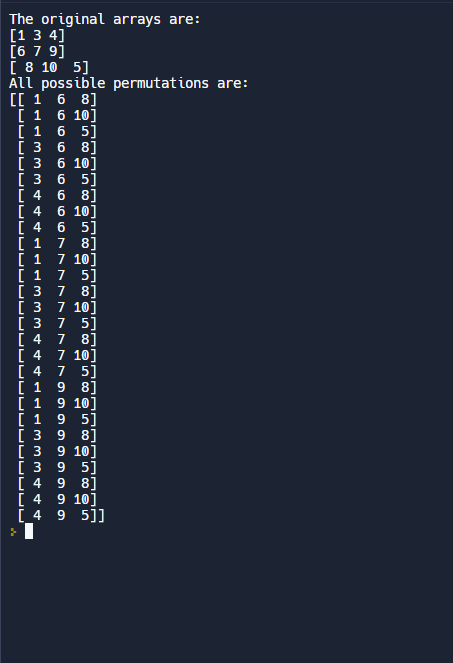# Python | All possible permutations of N lists

Computing permutations is always a necessary task in many of the practical applications and a concept widely used in Mathematics to achieve solutions to many practical problems. Lets discuss certain ways in which one can perform the task of getting all the permutations of N lists.

Method #1 : Using list comprehension List comprehension can be used to convert the naive method task into a single line, hence more compact. This method checks for each element available elements and makes pairs accordingly.

## Python3

 `# Python3 code to demonstrate` `# to compute all possible permutations` `# using list comprehension`   `# initializing lists` `list1 ``=` `[``1``, ``3``, ``4``]` `list2 ``=` `[``6``, ``7``, ``9``]` `list3 ``=` `[``8``, ``10``, ``5``]`   `# printing lists` `print` `(``"The original lists are : "` `+` `str``(list1) ``+` `                            ``" "` `+` `str``(list2) ``+` `                            ``" "` `+` `str``(list3))`   `# using list comprehension` `# to compute all possible permutations` `res ``=` `[[i, j, k] ``for` `i ``in` `list1` `                ``for` `j ``in` `list2` `                ``for` `k ``in` `list3]`   `# printing result` `print` `(``"All possible permutations are : "` `+` `str``(res))`

Output

```The original lists are : [1, 3, 4] [6, 7, 9] [8, 10, 5]
All possible permutations are : [[1, 6, 8], [1, 6, 10], [1, 6, 5], [1, 7, 8], [1, 7, 10], [1, 7, 5], [1, 9, 8], [1, 9, 10], [1, 9, 5], [3, 6, 8], [3, 6, 10], [3, 6, 5], [3, 7, 8], [3, 7, 10], [3, 7, 5], [3, 9, 8], [3, 9, 10], [3, 9, 5], [4, 6, 8], [4, 6, 10], [4, 6, 5], [4, 7, 8], [4, 7, 10], [4, 7, 5], [4, 9, 8], [4, 9, 10], [4, 9, 5]]
```

Time Complexity: O(n3) where n is the length of the list.
Space Complexity: O(n3) where n is the length of the list.

Method #2 : Using itertools.product() Using product function, one can easily perform this task in more pythonic and concise manner. This is most recommended method to perform this task of computing cartesian product.

## Python3

 `# Python3 code to demonstrate` `# to compute all possible permutations` `# using itertools.product()` `import` `itertools`   `# initializing list of list` `all_list ``=` `[[``1``, ``3``, ``4``], [``6``, ``7``, ``9``], [``8``, ``10``, ``5``] ]`   `# printing lists` `print` `(``"The original lists are : "` `+` `str``(all_list))`   `# using itertools.product()` `# to compute all possible permutations` `res ``=` `list``(itertools.product(``*``all_list))`   `# printing result` `print` `(``"All possible permutations are : "` `+` `str``(res))`

Output

```The original lists are : [[1, 3, 4], [6, 7, 9], [8, 10, 5]]
All possible permutations are : [(1, 6, 8), (1, 6, 10), (1, 6, 5), (1, 7, 8), (1, 7, 10), (1, 7, 5), (1, 9, 8), (1, 9, 10), (1, 9, 5), (3, 6, 8), (3, 6, 10), (3, 6, 5), (3, 7, 8), (3, 7, 10), (3, 7, 5), (3, 9, 8), (3, 9, 10), (3, 9, 5), (4, 6, 8), (4, 6, 10), (4, 6, 5), (4, 7, 8), (4, 7, 10), (4, 7, 5), (4, 9, 8), (4, 9, 10), (4, 9, 5)]
```

Time Complexity: O(nk) where n is the number of lists and k is the number of elements of each list.
Auxiliary Space: O(nk) where n is the number of lists and k is the number of elements of each list.

Method #3: Using numpy

In this method, numpy library is used to create a 3D array of all possible combinations of elements from the three lists using the np.meshgrid() function. The resulting 3D array is reshaped into a 2D array and stored in the res variable.

## Python3

 `import` `numpy as np`   `# initializing lists as arrays` `list1 ``=` `np.array([``1``, ``3``, ``4``])` `list2 ``=` `np.array([``6``, ``7``, ``9``])` `list3 ``=` `np.array([``8``, ``10``, ``5``])`   `# printing arrays` `print``(``"The original arrays are:"``)` `print``(list1)` `print``(list2)` `print``(list3)`   `# using NumPy meshgrid and stack` `# to compute all possible permutations` `X, Y, Z ``=` `np.meshgrid(list1, list2, list3)` `res ``=` `np.column_stack((X.ravel(), Y.ravel(), Z.ravel()))`   `# printing result` `print``(``"All possible permutations are:"``)` `print``(res)`   `# Contributed by rishabmalhdijo`

Output:Output

Time Complexity: O(n3) where n is the length of each list
Space Complexity: O(n3), because the resulting 3D array has to be stored in memory.

Whether you're preparing for your first job interview or aiming to upskill in this ever-evolving tech landscape, GeeksforGeeks Courses are your key to success. We provide top-quality content at affordable prices, all geared towards accelerating your growth in a time-bound manner. Join the millions we've already empowered, and we're here to do the same for you. Don't miss out - check it out now!

Previous
Next